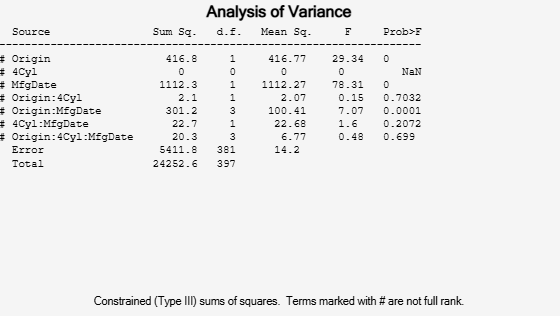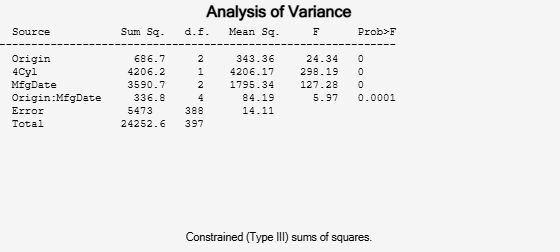## N-Way ANOVA

### Introduction to N-Way ANOVA

You can use the function `anovan` to perform N-way ANOVA. Use N-way ANOVA to determine if the means in a set of data differ with respect to groups (levels) of multiple factors. By default, `anovan` treats all grouping variables as fixed effects. For an example of ANOVA with random effects, see ANOVA with Random Effects. For repeated measures, see `fitrm` and `ranova`.

N-way ANOVA is a generalization of two-way ANOVA. For three factors, for example, the model can be written as

`${y}_{ijkr}=\mu +{\alpha }_{i}+{\beta }_{j}+{\gamma }_{k}+{\left(\alpha \beta \right)}_{ij}+{\left(\alpha \gamma \right)}_{ik}+{\left(\beta \gamma \right)}_{jk}+{\left(\alpha \beta \gamma \right)}_{ijk}+{\epsilon }_{ijkr,}$`

where

• yijkr is an observation of the response variable. i represents group i of factor A, i = 1, 2, ..., I, j represents group j of factor B, j = 1, 2, ..., J, k represents group k of factor C, and r represents the replication number, r = 1, 2, ..., R. For constant R, there are a total of N = I*J*K*R observations, but the number of observations does not have to be the same for each combination of groups of factors.

• μ is the overall mean.

• αi are the deviations of groups of factor A from the overall mean μ due to factor A. The values of αi sum to 0.

`${\sum }_{i=1}^{I}{\alpha }_{i}=0.$`

• βj are the deviations of groups in factor B from the overall mean μ due to factor B. The values of βj sum to 0.

`${\sum }_{j=1}^{J}{\beta }_{j}=0.$`

• γk are the deviations of groups in factor C from the overall mean μ due to factor C. The values of γk sum to 0.

`${\sum }_{k=1}^{K}{\gamma }_{k}=0.$`

• (αβ)ij is the interaction term between factors A and B. (αβ)ij sum to 0 over either index.

`${\sum }_{i=1}^{I}{\left(\alpha \beta \right)}_{ij}={\sum }_{j=1}^{J}{\left(\alpha \beta \right)}_{ij}=0.$`

• (αγ)ik is the interaction term between factors A and C. The values of (αγ)ik sum to 0 over either index.

`${\sum }_{i=1}^{I}{\left(\alpha \gamma \right)}_{ik}={\sum }_{k=1}^{K}{\left(\alpha \gamma \right)}_{ik}=0.$`

• (βγ)jk is the interaction term between factors B and C. The values of (βγ)jk sum to 0 over either index.

`${\sum }_{j=1}^{J}{\left(\beta \gamma \right)}_{jk}={\sum }_{k=1}^{K}{\left(\beta \gamma \right)}_{jk}=0.$`

• (αβγ)ijk is the three-way interaction term between factors A, B, and C. The values of (αβγ)ijk sum to 0 over any index.

`${\sum }_{i=1}^{I}{\left(\alpha \beta \gamma \right)}_{ijk}={\sum }_{j=1}^{J}{\left(\alpha \beta \gamma \right)}_{ijk}={\sum }_{k=1}^{K}{\left(\alpha \beta \gamma \right)}_{ijk}=0.$`

• εijkr are the random disturbances. They are assumed to be independent, normally distributed, and have constant variance.

Three-way ANOVA tests hypotheses about the effects of factors A, B, C, and their interactions on the response variable y. The hypotheses about the equality of the mean responses for groups of factor A are

The hypotheses about the equality of the mean response for groups of factor B are

The hypotheses about the equality of the mean response for groups of factor C are

The hypotheses about the interaction of the factors are

In this notation parameters with two subscripts, such as (αβ)ij, represent the interaction effect of two factors. The parameter (αβγ)ijk represents the three-way interaction. An ANOVA model can have the full set of parameters or any subset, but conventionally it does not include complex interaction terms unless it also includes all simpler terms for those factors. For example, one would generally not include the three-way interaction without also including all two-way interactions.

### Prepare Data for N-Way ANOVA

Unlike `anova1` and `anova2`, `anovan` does not expect data in a tabular form. Instead, it expects a vector of response measurements and a separate vector (or text array) containing the values corresponding to each factor. This input data format is more convenient than matrices when there are more than two factors or when the number of measurements per factor combination is not constant.

`$\begin{array}{ccccccccccc}y& =& \left[& {y}_{1},& {y}_{2},& {y}_{3},& {y}_{4},& {y}_{5},& \cdots ,& {y}_{N}& {\right]}^{\prime }\\ & & & ↑& ↑& ↑& ↑& ↑& & ↑& \\ g1& =& \left\{& \text{'}A\text{'},& \text{'}A\text{'},& \text{'}C\text{'},& \text{'}B\text{'},& \text{'}B\text{'},& \cdots ,& \text{'}D\text{'}& \right\}\\ g2& =& \left[& 1& 2& 1& 3& 1& \cdots ,& 2& \right]\\ g3& =& \left\{& \text{'}\text{hi}\text{'},& \text{'}\text{mid}\text{'},& \text{'}\text{low}\text{'},& \text{'}\text{mid}\text{'},& \text{'}\text{hi}\text{'},& \cdots ,& \text{'}\text{low}\text{'}& \right\}\end{array}$`

### Perform N-Way ANOVA

This example shows how to perform N-way ANOVA on car data with mileage and other information on 406 cars made between 1970 and 1982.

`load carbig`

The example focusses on four variables. `MPG` is the number of miles per gallon for each of 406 cars (though some have missing values coded as `NaN`). The other three variables are factors: `cyl4` (four-cylinder car or not), `org` (car originated in Europe, Japan, or the USA), and `when` (car was built early in the period, in the middle of the period, or late in the period).

Fit the full model, requesting up to three-way interactions and Type 3 sums-of-squares.

```varnames = {'Origin';'4Cyl';'MfgDate'}; anovan(MPG,{org cyl4 when},3,3,varnames)``````ans = 7×1 0.0000 NaN 0.0000 0.7032 0.0001 0.2072 0.6990 ```

Note that many terms are marked by a # symbol as not having full rank, and one of them has zero degrees of freedom and is missing a p-value. This can happen when there are missing factor combinations and the model has higher-order terms. In this case, the cross-tabulation below shows that there are no cars made in Europe during the early part of the period with other than four cylinders, as indicated by the 0 in `tbl(2,1,1)`.

`[tbl,chi2,p,factorvals] = crosstab(org,when,cyl4)`
```tbl = tbl(:,:,1) = 82 75 25 0 4 3 3 3 4 tbl(:,:,2) = 12 22 38 23 26 17 12 25 32 ```
```chi2 = 207.7689 ```
```p = 8.0973e-38 ```
```factorvals=3×3 cell array {'USA' } {'Early'} {'Other' } {'Europe'} {'Mid' } {'Four' } {'Japan' } {'Late' } {0x0 double} ```

Consequently it is impossible to estimate the three-way interaction effects, and including the three-way interaction term in the model makes the fit singular.

Using even the limited information available in the ANOVA table, you can see that the three-way interaction has a p-value of 0.699, so it is not significant.

Examine only two-way interactions.

`[p,tbl2,stats,terms] = anovan(MPG,{org cyl4 when},2,3,varnames);``terms`
```terms = 6×3 1 0 0 0 1 0 0 0 1 1 1 0 1 0 1 0 1 1 ```

Now all terms are estimable. The p-values for interaction term 4 (`Origin*4Cyl`) and interaction term 6 (`4Cyl*MfgDate`) are much larger than a typical cutoff value of 0.05, indicating these terms are not significant. You could choose to omit these terms and pool their effects into the error term. The output `terms` variable returns a matrix of codes, each of which is a bit pattern representing a term.

Omit terms from the model by deleting their entries from `terms`.

`terms([4 6],:) = []`
```terms = 4×3 1 0 0 0 1 0 0 0 1 1 0 1 ```

Run `anovan` again, this time supplying the resulting vector as the model argument. Also return the statistics required for multiple comparisons of factors.

`[~,~,stats] = anovan(MPG,{org cyl4 when},terms,3,varnames)````stats = struct with fields: source: 'anovan' resid: [3.1235 0.1235 3.1235 1.1235 2.1235 0.1235 -0.8765 ... ] coeffs: [18x1 double] Rtr: [10x10 double] rowbasis: [10x18 double] dfe: 388 mse: 14.1056 nullproject: [18x10 double] terms: [4x3 double] nlevels: [3x1 double] continuous: [0 0 0] vmeans: [3x1 double] termcols: [5x1 double] coeffnames: {18x1 cell} vars: [18x3 double] varnames: {3x1 cell} grpnames: {3x1 cell} vnested: [] ems: [] denom: [] dfdenom: [] msdenom: [] varest: [] varci: [] txtdenom: [] txtems: [] rtnames: [] ```

Now you have a more parsimonious model indicating that the mileage of these cars seems to be related to all three factors, and that the effect of the manufacturing date depends on where the car was made.

Perform multiple comparisons for Origin and Cylinder.

`results = multcompare(stats,'Dimension',[1,2])````results = 15×6 1.0000 2.0000 -5.4891 -3.8412 -2.1932 0.0000 1.0000 3.0000 -4.4146 -2.7251 -1.0356 0.0001 1.0000 4.0000 -9.9992 -8.5828 -7.1664 0 1.0000 5.0000 -14.0237 -12.4240 -10.8242 0 1.0000 6.0000 -12.8980 -11.3080 -9.7180 0 2.0000 3.0000 -0.7171 1.1160 2.9492 0.5085 2.0000 4.0000 -7.3655 -4.7417 -2.1179 0.0000 2.0000 5.0000 -9.9992 -8.5828 -7.1664 0 2.0000 6.0000 -9.7464 -7.4668 -5.1872 0.0000 3.0000 4.0000 -8.5396 -5.8577 -3.1757 0.0000 ⋮ ```